﻿ HTML5: How to require the current value of the gauge - w3resource

# HTML5: How to require the current value of the gauge?

Go to Exercise page

## Solution:

HTML Code:

``````<!DOCTYPE html>
<html>
<meta charset="utf-8">
<title>How to require the current value of the gauge</title>
<body>
<p>Display a gauge value here:</p>
<p>Harry's score: <meter min="0" low="20" high="80" max="100" value="90"></meter></p>
<p>James's score: <meter min="0" low="20" high="80" max="100" value="60"></meter></p>
<p>David's score: <meter min="0" low="20" high="80" max="100" value="30"></meter></p>
</body>
</html>
```
```

Live Demo:

See the Pen meter-value-answer by w3resource (@w3resource) on CodePen.

See the solution in the browser

Supported browser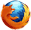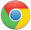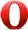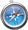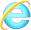Yes Yes Yes Yes Yes

Go to Exercise page

What is the difficulty level of this exercise?

Test your Programming skills with w3resource's quiz.

﻿